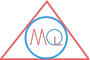# Area and Perimeter of Quadrilateral

## Introduction

As the number of sides of a quadrilateral are always fixed i.e. four, so, the types of Quadrilateral are classified based on how the length of four sides vary and how inclined the sides are.

## Perimeter

The perimeter of any plane figure is total length of its boundary. In other words, we can say perimeter is how long the boundary of a quadrilateral is and is calculated by adding up length of all sides of a quadrilateral. The perimeter is measured by same units as of length i.e. millimeter, centimeter, meter, kilometer etc.

Example

Example of perimeter calculation is how long a runner has to run around a rectangular shape playground to finish one round. The runner has to go around the boundary of the rectangle and cover up all the sides. So, the distance coverd by him will be equal to sum of all sides of rectangle, which is called as perimeter.

## Area

The area of any plane figure is the amount of surface enclosed by its sides. Let’s understand it by an example:

Example

Example of area calculation is how much area one has to paint on one side surface of a rectangular shape wooden board. If we need to paint both sides of rectangular board, then it will be two surfaces to paint that will make two areas of board to be painted.

Let’s have a look how to calculate area and perimeter of various types of quadrilaterals.

## Parallelogram

### Perimeter of parallelogram

Parallelogram with two parallel sides a, b and height hPerimeter of a parallelogram is calculated by adding up length of all sides.

The parallelogram in above figure has two parallel sides a, b and height h. We can write it as:

Perimeter $$= a + b + a + b$$ $$= 2a + 2b$$ $$= 2(a + b)$$

Formula

Perimeter of parallelogram $$= 2(a + b)$$

### Area of parallelogram

Area of a parallelogram is calculated by multiplying its length of base and height h.

So, area of parallelogram $$= base \times height$$ $$= b \times h$$

Formula

Area of parallelogram $$= b \times h$$

## Rectangle

### Perimeter of rectangle

Rectangle with length l and breadth bPerimeter of a rectangle is calculated by adding up the length of all sides. The rectangle in above figure has length l and breadth b. Therefore, the perimeter will be the sum of length of its four sides.

We can write it as:

Perimeter $$= l + b + l + b$$ $$= 2l + 2b$$ $$= 2(l + b)$$

Formula

Perimeter of rectangle $$= 2(l + b)$$

### Area of rectangle

Area of a rectangle is calculated by multiplying its length and breadth. So, area of square $$= length \times breadth$$

$$= l \times b$$

Formula

Area of square $$= l \times b$$

## Square

### Perimeter of square

Square with length l of its four sidesPerimeter of a square is calculated by adding up length of all sides.

The square in above figure has length l of its four sides. Therefore, the perimeter will be the sum of length of its four sides. We can write it as:

Perimeter $$= l + l + l + l = 4l$$

Perimeter of square can also be calculated by multiplying 4 with length l of square. So, perimeter can also be calculated as:

Perimeter $$= 4 \times l = 4l$$

Formula

Perimeter of square $$= 4l$$

### Area of square

Area of a square is calculated by multiplying its length and breadth.

So, area of square $$= length \times breadth$$ $$= l \times l$$ $$= l^{2}$$

Formula

Area of square $$= l^{2}$$

## Rhombus

### Perimeter of rhombus

Rhombus with length l and breadth bPerimeter of a rhombus is calculated by adding up length of all sides. The rhombus in above figure has length a of its all sides because length of all sides of a rhombus are equal always. Therefore, the perimeter will be the sum of length of its four sides. We can write it as:

Perimeter $$= a + a + a + a$$ $$= 4a$$

Perimeter of rhombus can also be calculated by multiplying 4 with length a. So, perimeter can also be calculated as:

Perimeter $$= 4 \times a$$ $$= 4a$$

Rhombus with diagonalsPerimeter of rhombus is also calculated using length of two diagonals.

Perimeter $$= 2\sqrt{d_1^{2} + d_2^{2}}$$

Formula

Perimeter of rhombus $$= 4a$$

Perimeter of rhombus $$= 2\sqrt{d_1^{2} + d_2^{2}}$$

### Area of rhombus

Area of a rhombus is calculated by multiplying its length of base and height h.

So, area of rhombus $$= base \times height$$ $$= a x h) Area of rhombus is also calculated using length of two diagonals. Area of rhombus \(= \frac{1}{2} \times d1 \times d2$$

Formula

Area of rhombus $$= a \times h$$

Area of rhombus $$= \frac{1}{2} \times d1 \times d2$$

## Trapezium

### Perimeter of trapezium

Trapezium with sides a, b, c, d and height hPerimeter of a trapezium is calculated by adding up length of all sides.

The trapezium in above figure has sides a, b, c, d and height h. We can write it as:

Perimeter $$= a + b + c + d$$

Formula

Perimeter of trapezium $$= a + b + c + d$$

### Area of trapezium

Area of trapezium $$= \frac{1}{2}(sum \; of \; parallel \; sides) \times h$$ $$= \frac{1}{2}(a + b) \times height$$

Formula

Area of trapezium $$= \frac{1}{2}(a + b) \times h$$